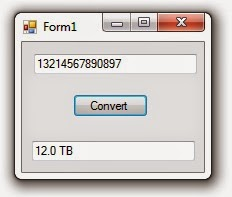# Convert Bytes to KB, MB, GB and TB in C#

`This c-sharp tutorial is about How to Convert Bytes or (Bits) to Kilobytes (KB) or Megabytes (MB) or Gigabytes (GB) or Terabytes (TB) or Petabytes (PB) or Yotabytes (YB) respectively in C#. In many cases specially where we are use filing in our application, it is likely to happen that we would need to represent the size of file in other formats than bytes. Here is my solution for it.`

## Source Code for Conversion in C#:

As always source code first.

### Output:

Here is the output of this code.### Explanation:

This method compares the value to larger and larger powers of 1024. When it finds a power of 1024 greater than or equal to the value, the code divides the value by the next smaller power and adds the appropriate suffix. For example, suppose the number is 10,000,000. This values is between 10242 = 1,048,576 and 10243 = 1,073,741,824. So the program divides the value by 10242 to get 10,000,000 ÷ 10242 ≈ 9.5361328125. It passes that value and the corresponding suffix MB to the following ThreeNonZeroDigits method. The ThreeNonZeroDigits method returns up to three non-zero digits for a number. If the value is at least 100, then it needs three digits anyway so the method simply returns the value formatted as a string with no values after the decimal point. If the value is at least 10 but less than 100, the method returns the value formatted as a string with two digits before the decimal point and one after. Finally if the value is less than 10, the method returns the value formatted as a string with one digit before the decimal point and two after.

### Note:

The ToFileSize method assumes a kilobyte is 1024 bytes. You can easily change this if you want to work with 1000 byte kilobytes.

### Fact:

As of 2011, the sum of the storage on every computer in the world was estimated at around 295 exabytes or 295 billion gigabytes, so this should be enough for a while, at least if you’re measuring real file sizes.